# ECE 576 POWER SYSTEM DYNAMICS AND STABILITY Lecture

• Slides: 27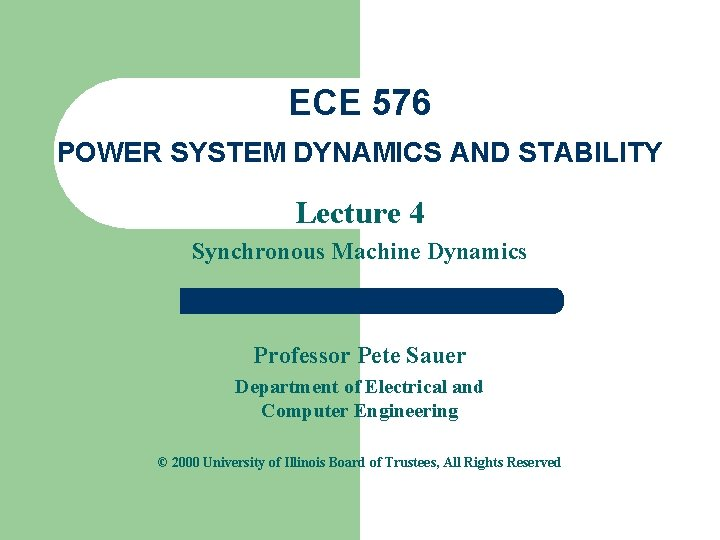ECE 576 POWER SYSTEM DYNAMICS AND STABILITY Lecture 4 Synchronous Machine Dynamics Professor Pete Sauer Department of Electrical and Computer Engineering © 2000 University of Illinois Board of Trustees, All Rights ReservedSynchronous machines 3 bal. windings (a, b, c) – stator Field winding (fd) on rotor Damper in “d” axis (1 d) on rotor 2 dampers in “q” axis (1 q, 2 q) on rotor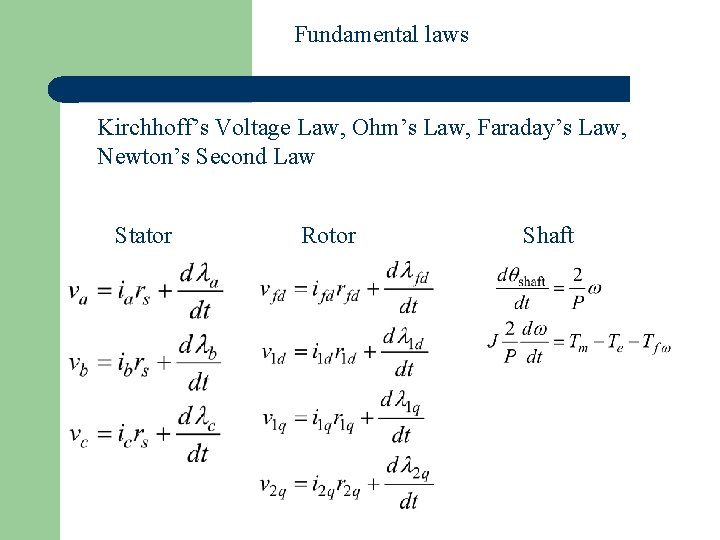Fundamental laws Kirchhoff’s Voltage Law, Ohm’s Law, Faraday’s Law, Newton’s Second Law Stator Rotor Shaft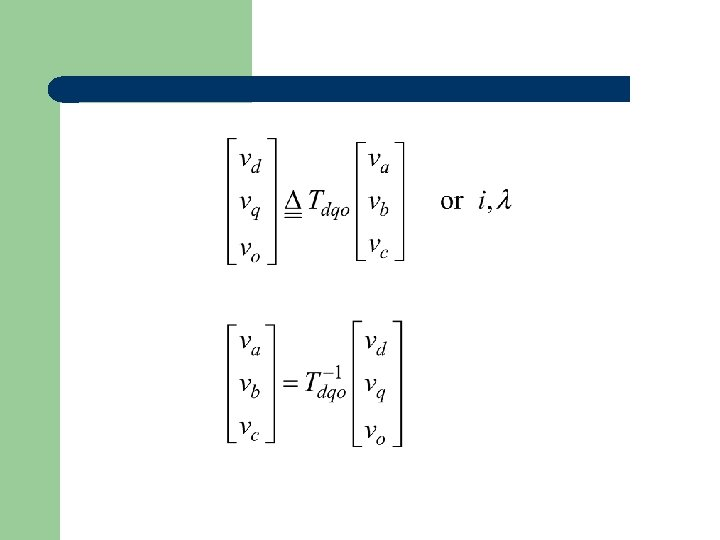with the inverse,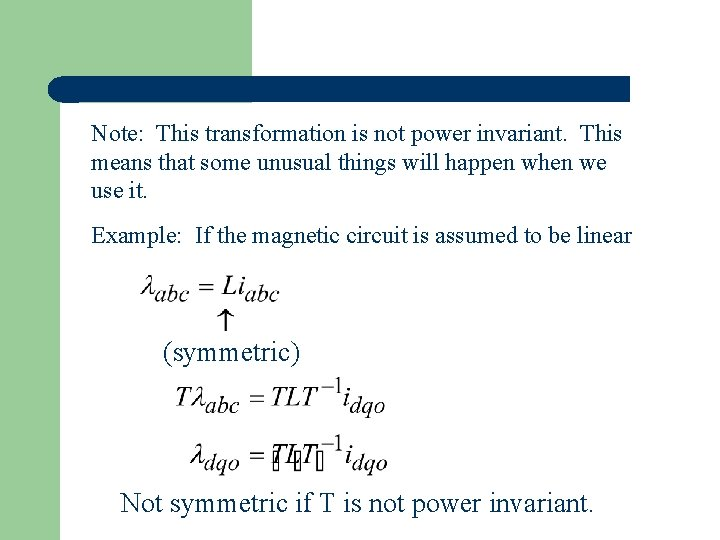Note: This transformation is not power invariant. This means that some unusual things will happen when we use it. Example: If the magnetic circuit is assumed to be linear (symmetric) Not symmetric if T is not power invariant.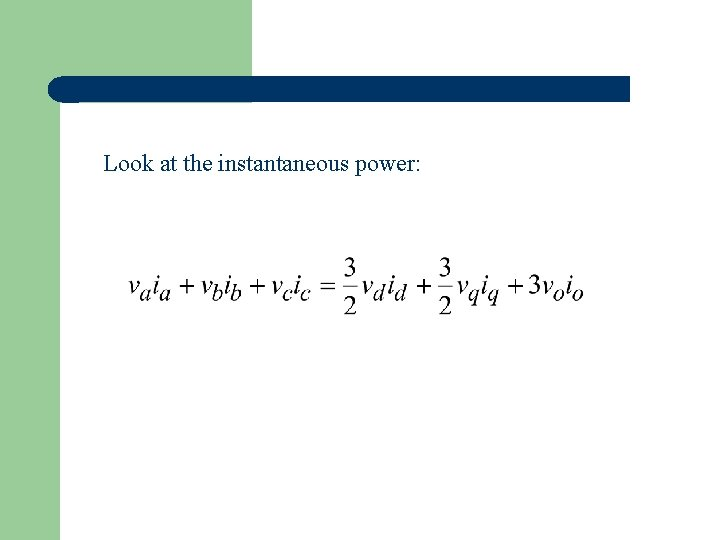Look at the instantaneous power:Transformed system Stator Rotor Shaft Use electrical angle:Energy conversion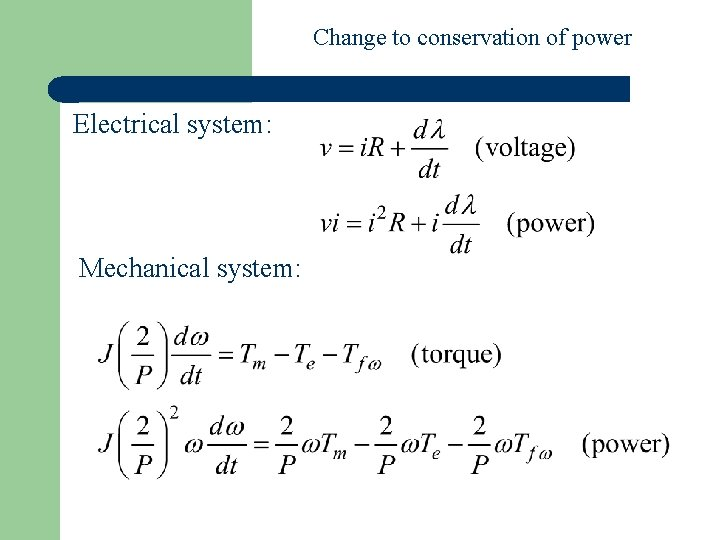Change to conservation of power Electrical system: Mechanical system: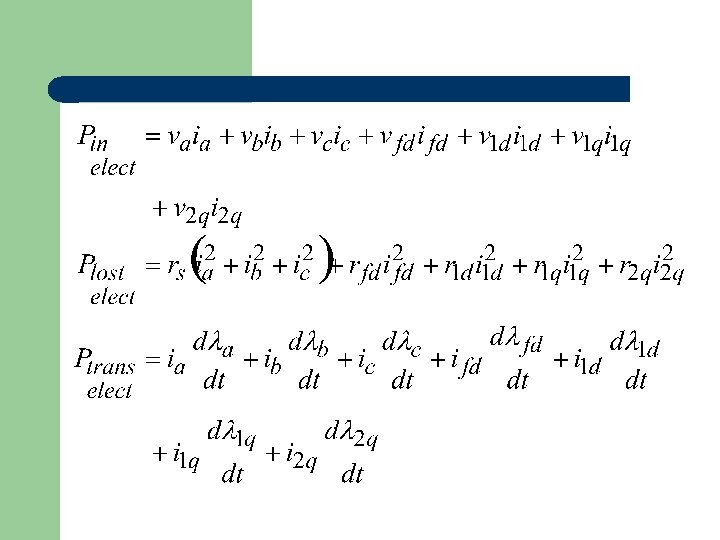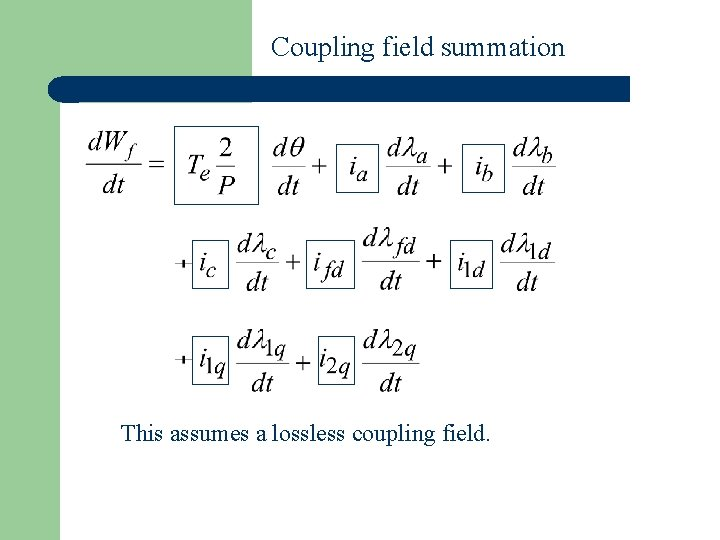Coupling field summation This assumes a lossless coupling field.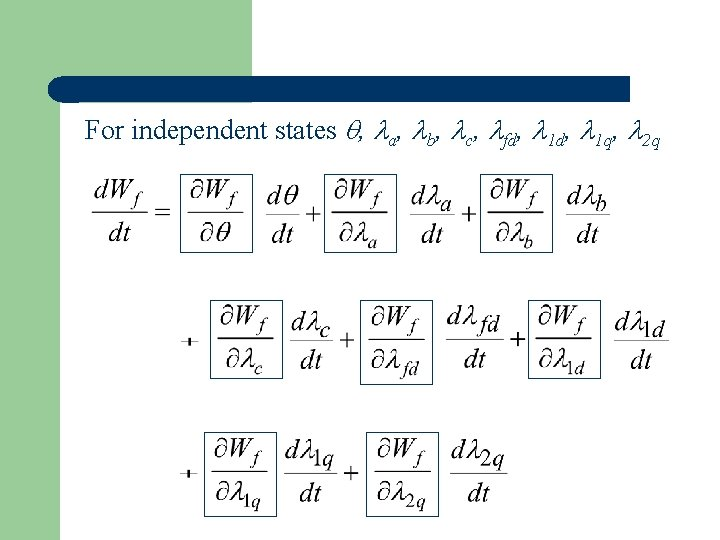For independent states , a, b, c, fd, 1 q, 2 q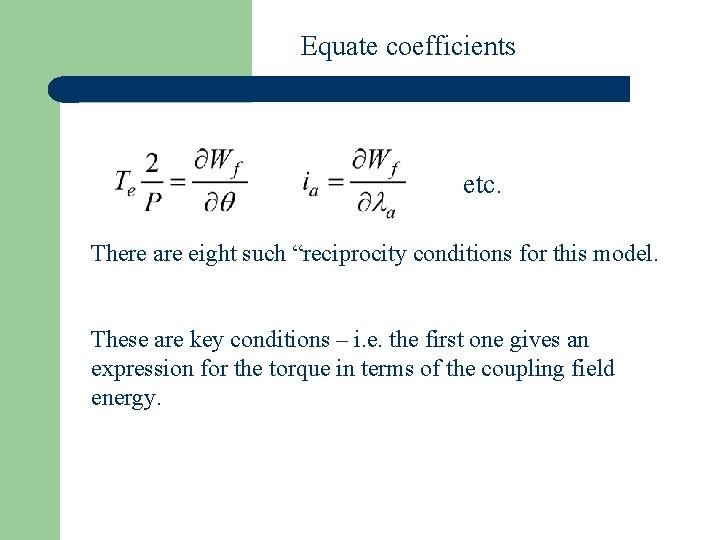Equate coefficients etc. There are eight such “reciprocity conditions for this model. These are key conditions – i. e. the first one gives an expression for the torque in terms of the coupling field energy.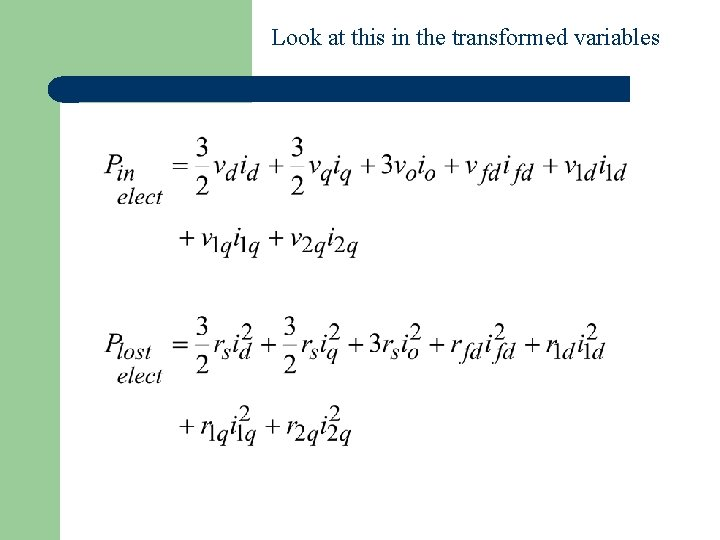Look at this in the transformed variables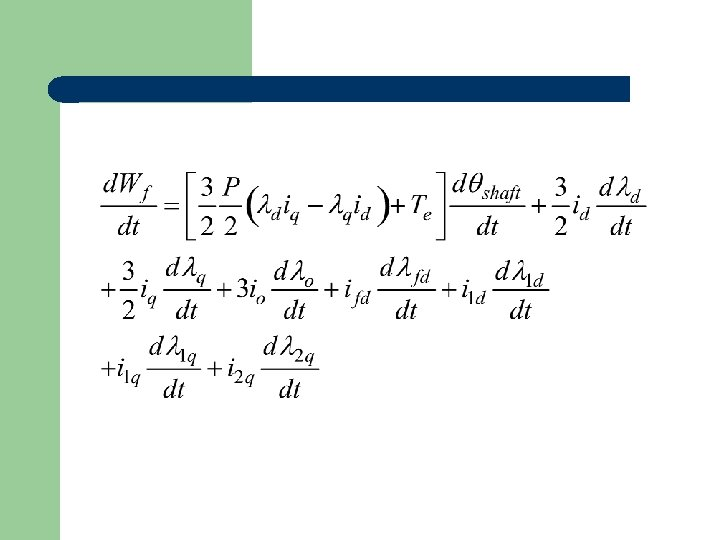For independent states shaft, d, q, o, fd, 1 d, 1 q, 2 qEquate coefficientsLook at second derivatives: This “non-symmetry” is due to the transformationWill show that: So that: Which gives us an expression for torque in terms of flux linkage and current (for linear or nonlinear magnetic model).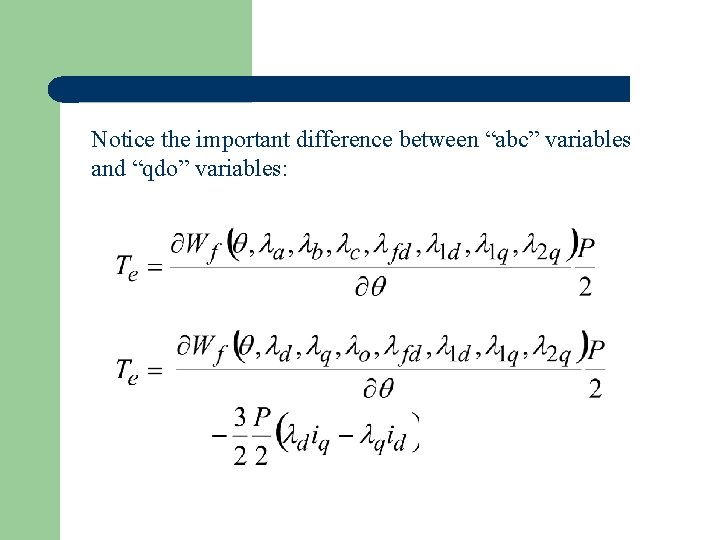Notice the important difference between “abc” variables and “qdo” variables:Change of variables in integration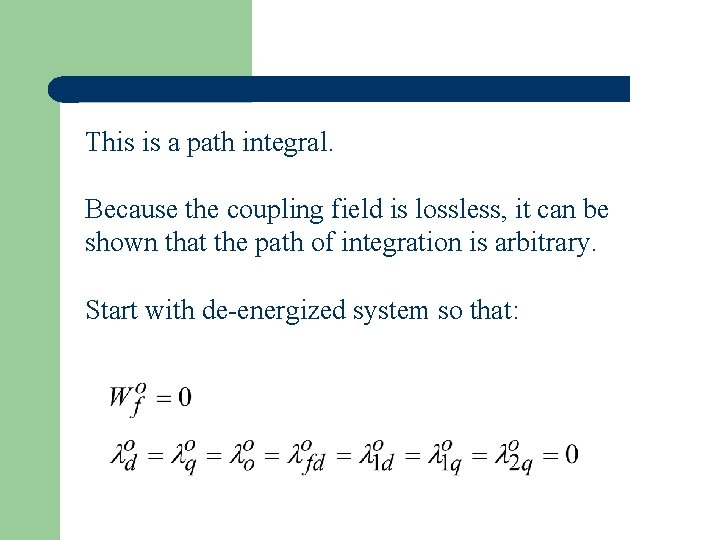This is a path integral. Because the coupling field is lossless, it can be shown that the path of integration is arbitrary. Start with de-energized system so that: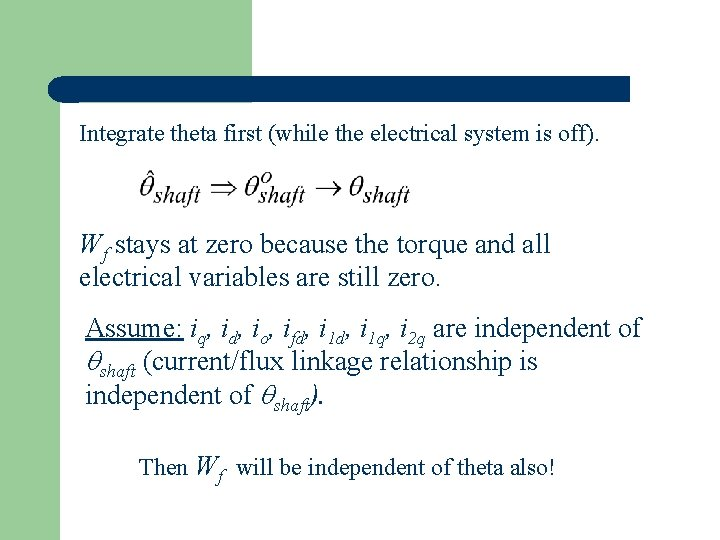Integrate theta first (while the electrical system is off). Wf stays at zero because the torque and all electrical variables are still zero. Assume: iq, id, io, ifd, i 1 q, i 2 q are independent of shaft (current/flux linkage relationship is independent of shaft). Then Wf will be independent of theta also!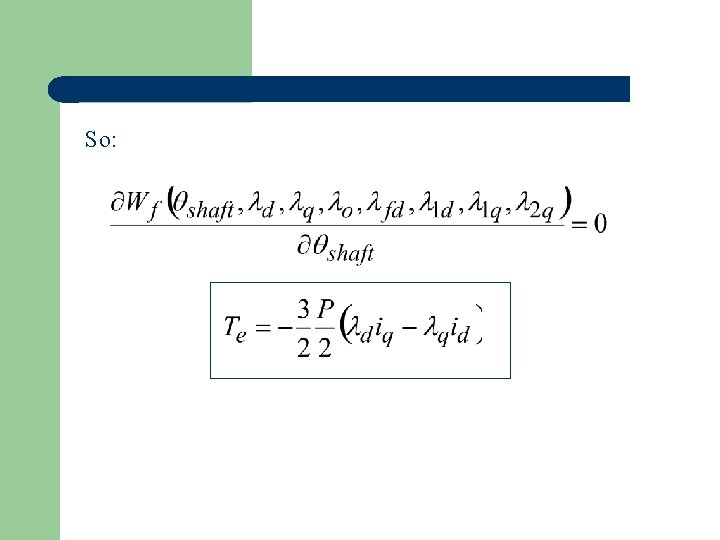So: